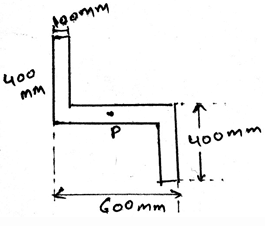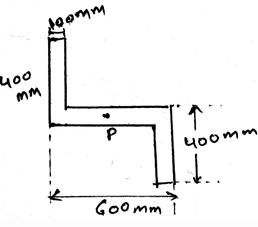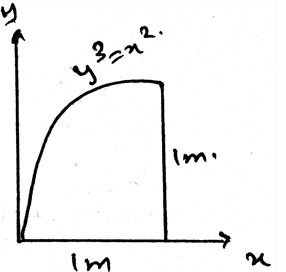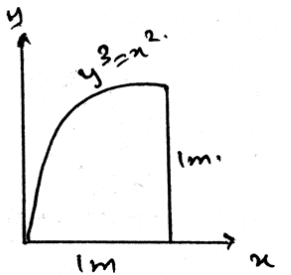Test: Moments Of Inertia For An Area About Inclined Axis

# Test: Moments Of Inertia For An Area About Inclined Axis

Test Description

## 30 Questions MCQ Test Additional Study Material for Mechanical Engineering | Test: Moments Of Inertia For An Area About Inclined Axis

Test: Moments Of Inertia For An Area About Inclined Axis for Mechanical Engineering 2023 is part of Additional Study Material for Mechanical Engineering preparation. The Test: Moments Of Inertia For An Area About Inclined Axis questions and answers have been prepared according to the Mechanical Engineering exam syllabus.The Test: Moments Of Inertia For An Area About Inclined Axis MCQs are made for Mechanical Engineering 2023 Exam. Find important definitions, questions, notes, meanings, examples, exercises, MCQs and online tests for Test: Moments Of Inertia For An Area About Inclined Axis below.
 1 Crore+ students have signed up on EduRev. Have you?
Test: Moments Of Inertia For An Area About Inclined Axis - Question 1

### If the non-Uniform loading is of the type of parabola then for calculating the moment of inertia for areas inclined at an axis?

Detailed Solution for Test: Moments Of Inertia For An Area About Inclined Axis - Question 1

The net force will act at the centroid of the parabola. Whether it be a parabola or the cubic curve the centroid is the only point at which the net force act. Force can’t be acted horizontally if the loading is vertical. Hence whatever be the shape of the loading, the centroid is the point of action of net force. Thus the use of centroid.

Test: Moments Of Inertia For An Area About Inclined Axis - Question 2

### Determine the orientation of the principle axis for the cross section of area of member shown whose width is 100mm.Detailed Solution for Test: Moments Of Inertia For An Area About Inclined Axis - Question 2

The net force will act at the centroid of the parabola. Whether it be a parabola or the cubic curve the centroid is the only point at which the net force act. Force can’t be acted horizontally if the loading is vertical. Hence whatever be the shape of the loading, the centroid is the point of action of net force. Thus the use of centroid.

Test: Moments Of Inertia For An Area About Inclined Axis - Question 3

### The product of Inertia for an area is required so as to__________

Detailed Solution for Test: Moments Of Inertia For An Area About Inclined Axis - Question 3

The product is required as to know what is the maximum and the minimum moment of inertia of an area. This data is required as to design the structure of the body. This means that the designing of the body is majorly done by the help of the determination of the product of the moment of Inertia.

Test: Moments Of Inertia For An Area About Inclined Axis - Question 4

Moment of Inertia about an inclined axis is the integration of the square of the distance of the centroid and the del area along the whole area of the structure and after this calculations we multiply the moment of areas.

Detailed Solution for Test: Moments Of Inertia For An Area About Inclined Axis - Question 4

The moment of inertia of the section is the integration of the square of the distance of the centroid and the del area along the whole area of the structure. This is having much significance in the various fields in the engineering sector. The main types are the ‘I’ section structures which are being much used.

Test: Moments Of Inertia For An Area About Inclined Axis - Question 5

The product of moment of inertia is the sum of _____________ and _________________. It can be used in the calculations of moments of inertia for an area about inclined axis.

Detailed Solution for Test: Moments Of Inertia For An Area About Inclined Axis - Question 5

The product of moment of inertia is required as to design the structure of the body. This means that the designing of the body is majorly done by the help of the determination of the product of the moment of Inertia.

Test: Moments Of Inertia For An Area About Inclined Axis - Question 6

There is perpendicular axis theorem for the area, and it is can be used to determine the moment of inertia of an area about inclined axis.

Detailed Solution for Test: Moments Of Inertia For An Area About Inclined Axis - Question 6

There is no perpendicular axis theorem for the area. In spite there is the theorem as parallel axis for any area. Thus we have the theorem which is used to add the two mutually perpendicular moment of inertias.

Test: Moments Of Inertia For An Area About Inclined Axis - Question 7

If any external force also is applied on the structure and we are determining the moment of inertia for areas about inclined axis then what should we consider?

Detailed Solution for Test: Moments Of Inertia For An Area About Inclined Axis - Question 7

The external forces are treated differently. They are not added by the force of the distributed loading. That is the force not only acts at the centroid always. It can be shifted also. Depending on the external forces. Thus the use of centroid or centre of mass.

Test: Moments Of Inertia For An Area About Inclined Axis - Question 8

Determine the magnitude of the principle moment of inertia for the cross section of area of member shown whose width is 100mm.Detailed Solution for Test: Moments Of Inertia For An Area About Inclined Axis - Question 8

The net force will act at the centroid of the parabola. Whether it be a parabola or the cubic curve the centroid is the only point at which the net force act. Force can’t be acted horizontally if the loading is vertical. Hence whatever be the shape of the loading, the centroid is the point of action of net force. Thus the use of centroid.

Test: Moments Of Inertia For An Area About Inclined Axis - Question 9

The calculation of moment of inertia about inclined axis due to the loadings involve a quantity called ____________

Detailed Solution for Test: Moments Of Inertia For An Area About Inclined Axis - Question 9

The calculation of the moment of the body due to the loadings involve a quantity called moment of inertia. This is having much significance in the various fields in the engineering sector. The main types are the ‘I’ section structures which are being much used.

Test: Moments Of Inertia For An Area About Inclined Axis - Question 10

Whenever the distributed loading acts perpendicular to an area its intensity varies __________ for the determination of moment of inertia about the inclined axis.

Detailed Solution for Test: Moments Of Inertia For An Area About Inclined Axis - Question 10

The load intensity is varying linearly in the structures. Thus the intensity is not varying parabolically nor is it cubically. It cannot be a vector also. Thus the intensity is linearly varied.

Test: Moments Of Inertia For An Area About Inclined Axis - Question 11

The distance in the parallel axis theorem for the use in the determination of the moment of inertia about an inclined axis is multiplied by:

Detailed Solution for Test: Moments Of Inertia For An Area About Inclined Axis - Question 11

Parallel axis for any area is used to add the two mutually perpendicular moment of inertias for areas. It gives a moment of inertia perpendicular to the surface of the body. And uses the square of the distance from the axis of rotation multiplied by the area.

Test: Moments Of Inertia For An Area About Inclined Axis - Question 12

One of the use of the centre of mass or centroid is as in the determination of the moment of inertia about an inclined plane is that the net force acts at the ___________ of the loading body.

Detailed Solution for Test: Moments Of Inertia For An Area About Inclined Axis - Question 12

In the moment of inertia calculations we see that the net force acts at the centroid of the loading body. That is if the loading system is in the form of the triangle then the at the distance 2 by 3 of the base the net force of the loading will act. And the load will be half the area of the loading.

Test: Moments Of Inertia For An Area About Inclined Axis - Question 13

What is parallel axis theorem and to whom it is applied so that it can give the product of inertia of an area of an area inclined about an axis?

Detailed Solution for Test: Moments Of Inertia For An Area About Inclined Axis - Question 13

Parallel axis for any area is used to add the two mutually perpendicular moment of inertias for areas. It gives a moment of inertia perpendicular to the surface of the body. That is the moment of inertia perpendicular to the surface in considerance.

Test: Moments Of Inertia For An Area About Inclined Axis - Question 14

The body is sometimes acted by two or three force members and we need to find the moment of inertia about the inclined axis for the same. The difference between the two and the three force members is:

Detailed Solution for Test: Moments Of Inertia For An Area About Inclined Axis - Question 14

The definition of the two force member only defines that the forces are being acted on the two points on the body. So does is the definition of the three forces members. The points of action of the three forces are three.

Test: Moments Of Inertia For An Area About Inclined Axis - Question 15

The parallel axis theorem can add any angle varied moment of inertias to give the perpendicular moment of inertia and it can be used in the determination of the moment of inertia about inclined axis.

Detailed Solution for Test: Moments Of Inertia For An Area About Inclined Axis - Question 15

Parallel axis for any area is used to add the two mutually perpendicular moment of inertias for areas. It gives a moment of inertia perpendicular to the surface of the body. That is the moment of inertia perpendicular to the surface in considerance.

Test: Moments Of Inertia For An Area About Inclined Axis - Question 16

In the calculations of the moment of inertia of the area about an inclined axis, we have some transformation done. They are:

Detailed Solution for Test: Moments Of Inertia For An Area About Inclined Axis - Question 16

In the moment of inertia calculations we see that the net force acts at the centroid of the loading body. That is if the loading system is in the form of the triangle then at the distance 2 by 3 of the base the net force of the loading will act. And the load will be half the area of the loading. Thus when there is the inclination of the axis, we use transformation.

Test: Moments Of Inertia For An Area About Inclined Axis - Question 17

In the calculations of moments of inertia for an area about inclined axis we use the product of moment of inertia. It is the the sum of _____________ and _________________

Detailed Solution for Test: Moments Of Inertia For An Area About Inclined Axis - Question 17

The product of moment of inertia is required as to design the structure of the body. This means that the designing of the body is majorly done by the help of the determination of the product of the moment of Inertia.

Test: Moments Of Inertia For An Area About Inclined Axis - Question 18

In the calculations of the moment of inertia of the area about an inclined axis, we have some transformation done. They are:

Detailed Solution for Test: Moments Of Inertia For An Area About Inclined Axis - Question 18

In the moment of inertia calculations we see that the net force acts at the centroid of the loading body. That is if the loading system is in the form of the triangle then at the distance 2 by 3 of the base the net force of the loading will act. And the load will be half the area of the loading. Thus when there is the inclination of the axis, we use transformation.

Test: Moments Of Inertia For An Area About Inclined Axis - Question 19

In the calculations of the moment of inertia of the area about an inclined axis, we have some transformation done. They are:

Detailed Solution for Test: Moments Of Inertia For An Area About Inclined Axis - Question 19

In the moment of inertia calculations we see that the net force acts at the centroid of the loading body. That is if the loading system is in the form of the triangle then at the distance 2 by 3 of the base the net force of the loading will act. And the load will be half the area of the loading. Thus when there is the inclination of the axis, we use transformation.

Test: Moments Of Inertia For An Area About Inclined Axis - Question 20

In the calculations of the moment of inertia of the area about an inclined axis, we have some transformation done. They are:

Detailed Solution for Test: Moments Of Inertia For An Area About Inclined Axis - Question 20

In the moment of inertia calculations we see that the net force acts at the centroid of the loading body. That is if the loading system is in the form of the triangle then at the distance 2 by 3 of the base the net force of the loading will act. And the load will be half the area of the loading. Thus when there is the inclination of the axis, we use transformation.

Test: Moments Of Inertia For An Area About Inclined Axis - Question 21

There is parallel axis theorem for the area, and it is can be used to determine the moment of inertia of an area about inclined axis.

Detailed Solution for Test: Moments Of Inertia For An Area About Inclined Axis - Question 21

There is no perpendicular axis theorem for the area. In spite there is the theorem as parallel axis for any area. Thus we have the theorem which is used to add the two mutually perpendicular moment of inertias.

Test: Moments Of Inertia For An Area About Inclined Axis - Question 22

Determine the moment of inertia of the area about y-axis.Detailed Solution for Test: Moments Of Inertia For An Area About Inclined Axis - Question 22

Parallel axis for any area is used to add the two mutually perpendicular moment of inertias for areas. It gives a moment of inertia perpendicular to the surface of the body. That is the moment of inertia perpendicular to the surface in considerance.

Test: Moments Of Inertia For An Area About Inclined Axis - Question 23

In the calculations of the moment of inertia of the area about an inclined axis, we have some transformation done. They are:

Detailed Solution for Test: Moments Of Inertia For An Area About Inclined Axis - Question 23

In the moment of inertia calculations we see that the net force acts at the centroid of the loading body. That is if the loading system is in the form of the triangle then at the distance 2 by 3 of the base the net force of the loading will act. And the load will be half the area of the loading. Thus when there is the inclination of the axis, we use transformation.

Test: Moments Of Inertia For An Area About Inclined Axis - Question 24

In the calculations of the moment of inertia of the area about an inclined axis, we have some transformation done. They are:

Detailed Solution for Test: Moments Of Inertia For An Area About Inclined Axis - Question 24

In the moment of inertia calculations we see that the net force acts at the centroid of the loading body. That is if the loading system is in the form of the triangle then at the distance 2 by 3 of the base the net force of the loading will act. And the load will be half the area of the loading. Thus when there is the inclination of the axis, we use transformation.

Test: Moments Of Inertia For An Area About Inclined Axis - Question 25

Moment of Inertia about an inclined axis is the integration of the cube of the distance of the centroid and the del area along the whole area of the structure and after this calculations we multiply the moment of areas.

Detailed Solution for Test: Moments Of Inertia For An Area About Inclined Axis - Question 25

The moment of inertia of the section is the integration of the square of the distance of the centroid and the del area along the whole area of the structure. This is having much significance in the various fields in the engineering sector. The main types are the ‘I’ section structures which are being much used.

Test: Moments Of Inertia For An Area About Inclined Axis - Question 26

Determine the moment of inertia of the area about x-axis.Detailed Solution for Test: Moments Of Inertia For An Area About Inclined Axis - Question 26

Parallel axis for any area is used to add the two mutually perpendicular moment of inertias for areas. It gives a moment of inertia perpendicular to the surface of the body. That is the moment of inertia perpendicular to the surface in considerance.

Test: Moments Of Inertia For An Area About Inclined Axis - Question 27

In the calculations of the moment of inertia of the area about an inclined axis, we have some transformation done. They are:

Detailed Solution for Test: Moments Of Inertia For An Area About Inclined Axis - Question 27

In the moment of inertia calculations we see that the net force acts at the centroid of the loading body. That is if the loading system is in the form of the triangle then at the distance 2 by 3 of the base the net force of the loading will act. And the load will be half the area of the loading. Thus when there is the inclination of the axis, we use transformation.

Test: Moments Of Inertia For An Area About Inclined Axis - Question 28

In the calculations of the moment of inertia of the area about an inclined axis, we have some transformation done. They are:

Detailed Solution for Test: Moments Of Inertia For An Area About Inclined Axis - Question 28

In the moment of inertia calculations we see that the net force acts at the centroid of the loading body. That is if the loading system is in the form of the triangle then at the distance 2 by 3 of the base the net force of the loading will act. And the load will be half the area of the loading. Thus when there is the inclination of the axis, we use transformation.

Test: Moments Of Inertia For An Area About Inclined Axis - Question 29

In the calculations of the moment of inertia of the area about an inclined axis, we have some transformation done. They are:

Detailed Solution for Test: Moments Of Inertia For An Area About Inclined Axis - Question 29

In the moment of inertia calculations we see that the net force acts at the centroid of the loading body. That is if the loading system is in the form of the triangle then at the distance 2 by 3 of the base the net force of the loading will act. And the load will be half the area of the loading. Thus when there is the inclination of the axis, we use transformation.

Test: Moments Of Inertia For An Area About Inclined Axis - Question 30

If any external moment along with the force is applied on the structure and we are determining the moment of inertia for areas about inclined axis then what should we consider?

Detailed Solution for Test: Moments Of Inertia For An Area About Inclined Axis - Question 30

The external forces are treated differently. They are not added by the force of the distributed loading. That is the force not only acts at the centroid always. It can be shifted also. Depending on the external forces. Thus the use of centroid or centre of mass.

## Additional Study Material for Mechanical Engineering

1 videos|30 docs|57 tests
 Use Code STAYHOME200 and get INR 200 additional OFF Use Coupon Code
Information about Test: Moments Of Inertia For An Area About Inclined Axis Page
In this test you can find the Exam questions for Test: Moments Of Inertia For An Area About Inclined Axis solved & explained in the simplest way possible. Besides giving Questions and answers for Test: Moments Of Inertia For An Area About Inclined Axis , EduRev gives you an ample number of Online tests for practice

## Additional Study Material for Mechanical Engineering

1 videos|30 docs|57 tests